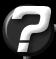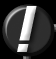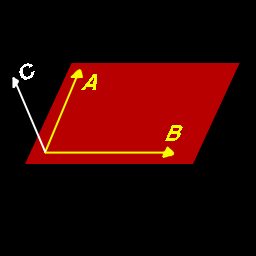Calculating Normals Question submitted by (23 July 1999)Return to The ArchivesDoes anyone know of a practical computer graphics book that explains the underlying maths understandably? I'm relatively new to graphics programming and the books I have seem to explain everything but what I need. The problem that I have is how to calculate the normal of a face given three vectors. Sorry if the question seems nieve, but if when you can't find somthing out yourself things grin to an abrupt halt.Vector maths are actually pretty simple once you get the hang of them. For me, the trick was to be able to visualize them. This extends nicely into matrices, since the standard rotational matrix (3x3) is simply three vectors (one for the X axis, one for the Y axis and one for the Z axis.)

As for your immediate problem (calculating the normal of a polygon given three vertices) the answer is quite simple. You perform a cross-product of two "edge vectors." Don't run away just yet, I'm about to explain this in simpler terms.

A cross product of two vectors returns another vector. The unique property about the cross product, is that it returns the normal of the plane that is defined by the two input vectors.In Figure 1, the vectors A & B define a plane in 3-space. These vectors were drawn such that they meet at a center axis, but this really isn't necessary, it just makes for prettier artwork (if you can call it that.)

By performing a cross-product on them, you end up with vector C. Here's what a cross-product looks like:

 ``` C.x = (A.y * B.z) - (A.z * B.y); C.y = (A.z * B.x) - (A.x * B.z); C.z = (A.x * B.y) - (A.y * a.x); ```

A triangle is usually defined by three vertices. These vertices are all connected together by edges. But you can think of each of these edges as a vector:In the above figure, edge a is defined by the subtraction of vertex B from vertex A, therefore the subtraction of any two points results in a vector. If you continue the chain around the polygon, you end up with three 'edge vectors.' This subtraction is very simple:

 ``` v.x = point2.x - point1.x; v.y = point2.y - point1.y; v.z = point2.z - point1.z; ```

The order of the subtraction determines the signed direction of the vector.

By now, you've probably already put it all together, but I'll conclude anyway. By subtracting vertex B from vertex A to get one vector, then subtract vertex C from vertex B to get the second vector, you have the information required for a cross product.

After performing the cross product, you'll have a vector that defines the surface normal. You will probably want to normalize this resulting normal vector. A vector normalization looks like this:

 ``` double vectorLength = sqrt(v.x * v.x + v.y * v.y + v.z * v.z); v.x /= length; v.y /= length; v.z /= length; ```

The vector length is much like calculating the length of a line. However, rather than having two end-points, a vector has only a single point. You can consider that point to be the second end-point, with the first end-point at the origin (0,0,0). Given that, you can see that you don't need the subtraction of the first point, since subtracting zero is useless. By dividing each element in the vector by it's length, you end up with a unit vector (a vector whose length is 1.0). Anything divided its length is 1.0.Response provided by Paul NettleThis article was originally an entry in flipCode's Fountain of Knowledge, an open Question and Answer column that no longer exists.